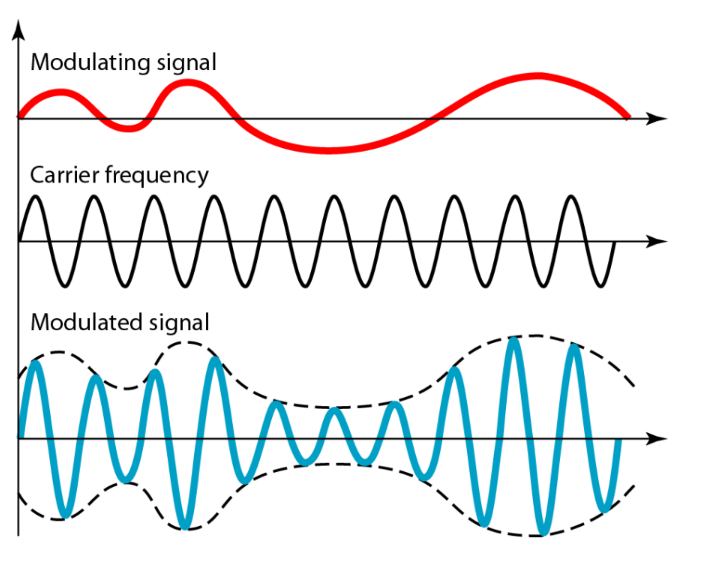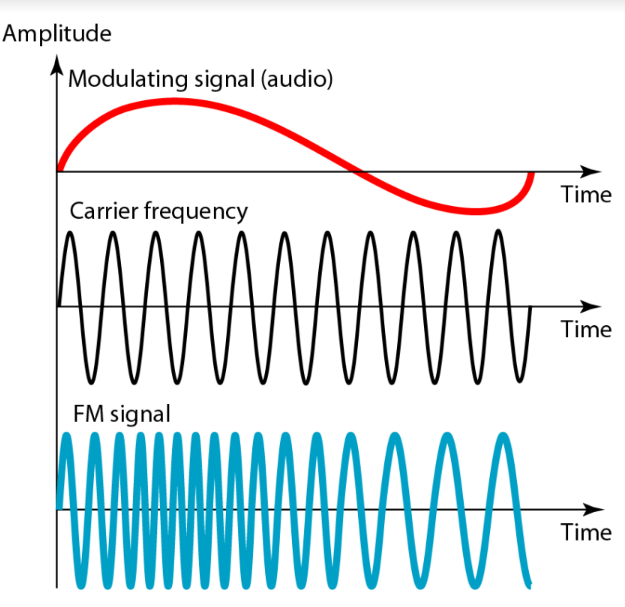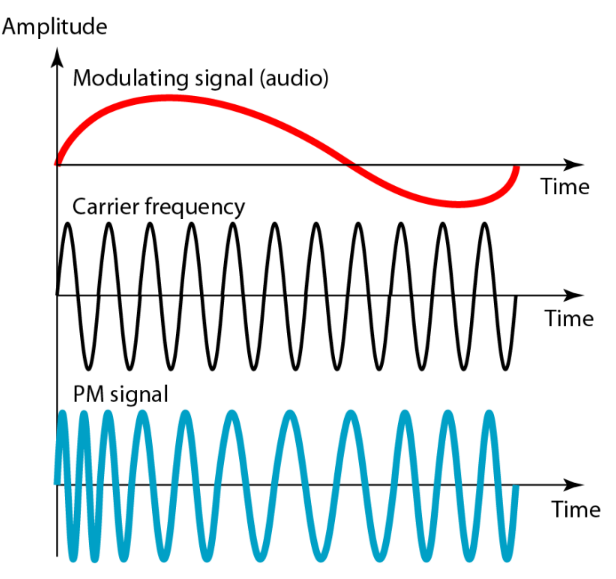×

Search anything:

# Overview of Analog CommunicationGet this book -> Problems on Array: For Interviews and Competitive Programming

In this article at OpenGenus, we will go through an overview of Analog Communication.

1. Modulation
2. Analog to analog conversion
3. Types of analog to analog conversion
4. Amplitude Modulation (AM)
5. Applications of Amplitude modulation
6. Frequency modulation (FM)
7. Applications of Amplitude modulation
8. Phase Modulation (PM)
9. Applications of Phase Modulation

# Modulation

• Superimposition of a low-frequency signal on a high-frequency carrier signal.

# Analog to analog conversion

• Analog signal is modulated if the medium is bandpass(i.e. it allows only a certain range/ frequencies of electric waves and blocks the others).

# Types of analog to analog conversion

• Amplitude modulation
• Frequency modulation
• Phase modulation

# Amplitude Modulation (AM)

• Base band/modulating signal which is a continuous wave containing information needs to be modulated.
• The amplitude of the carrier signal(containing no information) varies in comparison to the instantaneous amplitude of the modulating signal(contains information).• The first figure shows the modulating wave, which carries the information.
• The second one is the carrier wave, which is a high frequency signal and contains no information.
• The last one is the modulated wave, which is the result of superposition of modulating and carrier signal.
• The total bandwidth of the modulated signal can be determined by the bandwidth of audio signal which is 2B(where B is the bandwidth of modulating signal).

## Applications of Amplitude modulation

• Communication between Aircraft: Amplitude modulation is used for pilot-to-ground control communications other than techniques like frequency modulation because it can receive numerous signals on the same channel.
• Broadcasting: Mostly used for medium wave transmissions, but also on the longwave and shortwave radio bands.

# Frequency modulation (FM)

• The carrier wave frequency is varied in comparison with the frequency of the modulating signal.
• The approach of frequency modulation is quite similar to amplitude modulation just that in FM, amplitude is constant.• The first figure shows the modulating wave, which carries the information.
• The second one is the carrier wave, which is a high frequency signal and contains no information.
• The last one is the modulated wave, which is the result of superposition of modulating and carrier signal.
• The total bandwidth of the modulated signal can be determined by the bandwidth of audio signal which is 2(1+β)B(where B is the bandwidth of modulating signal, β is modulation index and is usually 4).

## Applications of Amplitude modulation

• Radio broadcasting: FM has a larger signal-to-noise ratio, which means it will reject radio frequency interferences much better than an equal power amplitude modulation (AM) signal. Due to this, most music is broadcasted over FM radio.

# Phase Modulation (PM)

• Phase of the carrier wave is varies whereas the amplitude is unchanged.
• Frequency also varies.• The sinusoidal signal starts to increase and reaches the maximum value, then the phase of the carrier signal increases.
• Due to this, a compression in the carrier signal is noticed. This hence increases the frequency of the signal.
• The total bandwidth of the modulated signal can be determined by the bandwidth of audio signal which is 2(1+β)B(where B is the bandwidth of modulating signal, β is modulation index and is usually 2).

## Applications of Phase Modulation

• GPS: In Global Positioning System (GPS) , a modulated signal is transmitted from a satellite, and the signal is received by a GPS receiver on the ground. By analyzing the phase of the received signal is analyzed and then the GPS receiver determines the distance between the satellite and the receiver, which is used to calculate the receiver's location.